# Mean State

Period Mean (original grids) [Watt m-2]
Model Period Mean (intersection) [Watt m-2]
Model Period Mean (complement) [Watt m-2]
Benchmark Period Mean (intersection) [Watt m-2]
Benchmark Period Mean (complement) [Watt m-2]
Bias [Watt m-2]
RMSE [Watt m-2]
Phase Shift [months]
Bias Score 
RMSE Score 
Seasonal Cycle Score 
Spatial Distribution Score 
Interannual Variability Score 
Overall Score 
Benchmark [-] -56.8
CLM4 [-] -51.3 -51.0 0.00 -57.0 -52.3 6.43 15.0 2.14 0.51 0.46 0.67 0.98 0.57 0.61
CLM4.5 [-] -55.3 -55.3 0.00 -57.0 -52.3 2.36 15.8 2.17 0.49 0.45 0.67 0.98 0.57 0.60
CLM5 [-] -56.5 -56.4 0.00 -57.0 -52.3 1.23 14.0 2.12 0.59 0.46 0.68 0.99 0.55 0.62
Period Mean (original grids) [Watt m-2]
Model Period Mean (intersection) [Watt m-2]
Model Period Mean (complement) [Watt m-2]
Benchmark Period Mean (intersection) [Watt m-2]
Benchmark Period Mean (complement) [Watt m-2]
Bias [Watt m-2]
RMSE [Watt m-2]
Phase Shift [months]
Bias Score 
RMSE Score 
Seasonal Cycle Score 
Spatial Distribution Score 
Interannual Variability Score 
Overall Score 
Benchmark [-] -87.0
CLM4 [-] -83.4 -84.3 0.00 -87.4 -58.4 3.55 18.6 0.234 0.80 0.58 0.98 0.99 0.57 0.75
CLM4.5 [-] -88.6 -89.3 0.00 -87.4 -58.4 -1.45 19.5 0.294 0.75 0.59 0.97 0.88 0.57 0.72
CLM5 [-] -90.0 -90.7 0.00 -87.4 -58.4 -2.82 19.4 0.297 0.76 0.58 0.97 0.91 0.53 0.72
Period Mean (original grids) [Watt m-2]
Model Period Mean (intersection) [Watt m-2]
Model Period Mean (complement) [Watt m-2]
Benchmark Period Mean (intersection) [Watt m-2]
Benchmark Period Mean (complement) [Watt m-2]
Bias [Watt m-2]
RMSE [Watt m-2]
Phase Shift [months]
Bias Score 
RMSE Score 
Seasonal Cycle Score 
Spatial Distribution Score 
Interannual Variability Score 
Overall Score 
Benchmark [-] -81.4
CLM4 [-] -77.1 -77.3 0.00 -82.3 -62.6 6.74 19.5 1.50 0.68 0.49 0.78 1.0 0.53 0.66
CLM4.5 [-] -82.8 -83.0 0.00 -82.3 -62.6 1.65 19.8 1.46 0.68 0.48 0.79 0.99 0.54 0.66
CLM5 [-] -80.9 -81.1 0.00 -82.3 -62.6 3.32 20.0 1.18 0.70 0.47 0.85 0.99 0.50 0.67
Period Mean (original grids) [Watt m-2]
Model Period Mean (intersection) [Watt m-2]
Model Period Mean (complement) [Watt m-2]
Benchmark Period Mean (intersection) [Watt m-2]
Benchmark Period Mean (complement) [Watt m-2]
Bias [Watt m-2]
RMSE [Watt m-2]
Phase Shift [months]
Bias Score 
RMSE Score 
Seasonal Cycle Score 
Spatial Distribution Score 
Interannual Variability Score 
Overall Score 
Benchmark [-] -37.2
CLM4 [-] -52.3 -52.3 0.00 -36.9 -41.0 -15.6 20.2 1.04 0.39 0.47 0.88 0.81 0.57 0.60
CLM4.5 [-] -54.1 -54.1 0.00 -36.9 -41.0 -17.0 21.0 1.07 0.36 0.49 0.88 0.93 0.54 0.61
CLM5 [-] -60.3 -60.4 0.00 -36.9 -41.0 -23.3 26.1 1.03 0.24 0.49 0.88 0.83 0.50 0.57
Period Mean (original grids) [Watt m-2]
Model Period Mean (intersection) [Watt m-2]
Model Period Mean (complement) [Watt m-2]
Benchmark Period Mean (intersection) [Watt m-2]
Benchmark Period Mean (complement) [Watt m-2]
Bias [Watt m-2]
RMSE [Watt m-2]
Phase Shift [months]
Bias Score 
RMSE Score 
Seasonal Cycle Score 
Spatial Distribution Score 
Interannual Variability Score 
Overall Score 
Benchmark [-] -44.6
CLM4 [-] -23.1 -23.1 0.00 -43.2 -49.2 20.4 21.9 3.04 0.067 0.36 0.49 0.86 0.56 0.45
CLM4.5 [-] -24.7 -24.7 0.00 -43.2 -49.2 19.3 21.0 3.04 0.075 0.37 0.49 0.88 0.55 0.45
CLM5 [-] -29.4 -29.3 0.00 -43.2 -49.2 14.9 17.2 2.67 0.11 0.37 0.55 0.88 0.52 0.47
Period Mean (original grids) [Watt m-2]
Model Period Mean (intersection) [Watt m-2]
Model Period Mean (complement) [Watt m-2]
Benchmark Period Mean (intersection) [Watt m-2]
Benchmark Period Mean (complement) [Watt m-2]
Bias [Watt m-2]
RMSE [Watt m-2]
Phase Shift [months]
Bias Score 
RMSE Score 
Seasonal Cycle Score 
Spatial Distribution Score 
Interannual Variability Score 
Overall Score 
Benchmark [-] -42.1
CLM4 [-] -59.0 -58.9 0.00 -41.9 -51.7 -17.0 20.4 0.853 0.35 0.51 0.91 0.80 0.56 0.60
CLM4.5 [-] -59.4 -59.4 0.00 -41.9 -51.7 -17.5 20.9 0.897 0.34 0.50 0.90 0.90 0.58 0.62
CLM5 [-] -65.8 -65.8 0.00 -41.9 -51.7 -23.9 27.0 1.08 0.23 0.46 0.87 0.76 0.54 0.55
Period Mean (original grids) [Watt m-2]
Model Period Mean (intersection) [Watt m-2]
Model Period Mean (complement) [Watt m-2]
Benchmark Period Mean (intersection) [Watt m-2]
Benchmark Period Mean (complement) [Watt m-2]
Bias [Watt m-2]
RMSE [Watt m-2]
Phase Shift [months]
Bias Score 
RMSE Score 
Seasonal Cycle Score 
Spatial Distribution Score 
Interannual Variability Score 
Overall Score 
Benchmark [-] -67.1
CLM4 [-] -77.1 -76.9 0.00 -67.3 -59.4 -8.57 19.3 1.13 0.58 0.48 0.85 0.97 0.56 0.65
CLM4.5 [-] -77.8 -77.7 0.00 -67.3 -59.4 -9.28 19.4 1.14 0.57 0.49 0.85 0.97 0.56 0.65
CLM5 [-] -82.2 -82.1 0.00 -67.3 -59.4 -13.7 22.2 1.16 0.48 0.47 0.84 0.94 0.54 0.62
Period Mean (original grids) [Watt m-2]
Model Period Mean (intersection) [Watt m-2]
Model Period Mean (complement) [Watt m-2]
Benchmark Period Mean (intersection) [Watt m-2]
Benchmark Period Mean (complement) [Watt m-2]
Bias [Watt m-2]
RMSE [Watt m-2]
Phase Shift [months]
Bias Score 
RMSE Score 
Seasonal Cycle Score 
Spatial Distribution Score 
Interannual Variability Score 
Overall Score 
Benchmark [-] -71.2
CLM4 [-] -66.5 -66.6 0.00 -72.6 -53.4 10.3 21.4 1.06 0.59 0.52 0.87 0.98 0.54 0.67
CLM4.5 [-] -70.7 -70.8 0.00 -72.6 -53.4 6.52 20.6 1.05 0.64 0.52 0.87 0.98 0.54 0.68
CLM5 [-] -72.7 -72.7 0.00 -72.6 -53.4 4.54 20.1 1.01 0.66 0.52 0.87 0.99 0.49 0.67
Period Mean (original grids) [Watt m-2]
Model Period Mean (intersection) [Watt m-2]
Model Period Mean (complement) [Watt m-2]
Benchmark Period Mean (intersection) [Watt m-2]
Benchmark Period Mean (complement) [Watt m-2]
Bias [Watt m-2]
RMSE [Watt m-2]
Phase Shift [months]
Bias Score 
RMSE Score 
Seasonal Cycle Score 
Spatial Distribution Score 
Interannual Variability Score 
Overall Score 
Benchmark [-] -31.1
CLM4 [-] -50.4 -50.5 0.00 -31.1 -32.1 -19.4 22.2 0.796 0.30 0.51 0.93 0.98 0.58 0.64
CLM4.5 [-] -50.6 -50.7 0.00 -31.1 -32.1 -19.5 22.2 0.817 0.30 0.51 0.93 1.0 0.58 0.64
CLM5 [-] -58.4 -58.5 0.00 -31.1 -32.1 -27.2 29.3 0.789 0.18 0.50 0.93 0.96 0.53 0.60
Period Mean (original grids) [Watt m-2]
Model Period Mean (intersection) [Watt m-2]
Model Period Mean (complement) [Watt m-2]
Benchmark Period Mean (intersection) [Watt m-2]
Benchmark Period Mean (complement) [Watt m-2]
Bias [Watt m-2]
RMSE [Watt m-2]
Phase Shift [months]
Bias Score 
RMSE Score 
Seasonal Cycle Score 
Spatial Distribution Score 
Interannual Variability Score 
Overall Score 
Benchmark [-] -96.8
CLM4 [-] -94.4 -94.5 0.00 -99.4 -63.9 8.01 18.9 0.574 0.60 0.52 0.91 0.89 0.54 0.67
CLM4.5 [-] -94.7 -94.7 0.00 -99.4 -63.9 7.72 18.8 0.566 0.61 0.52 0.92 0.88 0.55 0.67
CLM5 [-] -96.7 -96.7 0.00 -99.4 -63.9 5.96 19.5 0.614 0.61 0.51 0.91 0.91 0.51 0.66
Period Mean (original grids) [Watt m-2]
Model Period Mean (intersection) [Watt m-2]
Model Period Mean (complement) [Watt m-2]
Benchmark Period Mean (intersection) [Watt m-2]
Benchmark Period Mean (complement) [Watt m-2]
Bias [Watt m-2]
RMSE [Watt m-2]
Phase Shift [months]
Bias Score 
RMSE Score 
Seasonal Cycle Score 
Spatial Distribution Score 
Interannual Variability Score 
Overall Score 
Benchmark [-] -55.6
CLM4 [-] -66.3 -66.3 0.00 -55.1 -60.8 -10.9 16.9 1.03 0.40 0.46 0.87 0.70 0.60 0.58
CLM4.5 [-] -65.7 -65.7 0.00 -55.1 -60.8 -10.3 16.4 1.08 0.41 0.47 0.86 0.64 0.58 0.57
CLM5 [-] -70.2 -70.3 0.00 -55.1 -60.8 -14.6 19.5 1.21 0.34 0.46 0.83 0.55 0.53 0.53
Period Mean (original grids) [Watt m-2]
Model Period Mean (intersection) [Watt m-2]
Model Period Mean (complement) [Watt m-2]
Benchmark Period Mean (intersection) [Watt m-2]
Benchmark Period Mean (complement) [Watt m-2]
Bias [Watt m-2]
RMSE [Watt m-2]
Phase Shift [months]
Bias Score 
RMSE Score 
Seasonal Cycle Score 
Spatial Distribution Score 
Interannual Variability Score 
Overall Score 
Benchmark [-] -52.6
CLM4 [-] -66.8 -66.8 0.00 -65.2 -46.5 -0.108 20.6 1.25 0.49 0.48 0.83 0.92 0.53 0.62
CLM4.5 [-] -68.3 -68.3 0.00 -65.2 -46.5 -1.39 20.4 1.21 0.49 0.48 0.83 0.93 0.53 0.62
CLM5 [-] -71.7 -71.7 0.00 -65.2 -46.5 -4.65 22.3 1.23 0.45 0.47 0.83 0.91 0.50 0.61
Period Mean (original grids) [Watt m-2]
Model Period Mean (intersection) [Watt m-2]
Model Period Mean (complement) [Watt m-2]
Benchmark Period Mean (intersection) [Watt m-2]
Benchmark Period Mean (complement) [Watt m-2]
Bias [Watt m-2]
RMSE [Watt m-2]
Phase Shift [months]
Bias Score 
RMSE Score 
Seasonal Cycle Score 
Spatial Distribution Score 
Interannual Variability Score 
Overall Score 
Benchmark [-] -121.
CLM4 [-] -102. -102. 0.00 -122. -81.3 21.4 32.1 0.870 0.49 0.48 0.89 0.80 0.44 0.60
CLM4.5 [-] -102. -102. 0.00 -122. -81.3 21.5 32.1 0.879 0.48 0.48 0.89 0.81 0.44 0.60
CLM5 [-] -103. -103. 0.00 -122. -81.3 20.0 32.1 0.847 0.50 0.47 0.89 0.80 0.43 0.59
Period Mean (original grids) [Watt m-2]
Model Period Mean (intersection) [Watt m-2]
Model Period Mean (complement) [Watt m-2]
Benchmark Period Mean (intersection) [Watt m-2]
Benchmark Period Mean (complement) [Watt m-2]
Bias [Watt m-2]
RMSE [Watt m-2]
Phase Shift [months]
Bias Score 
RMSE Score 
Seasonal Cycle Score 
Spatial Distribution Score 
Interannual Variability Score 
Overall Score 
Benchmark [-] -34.3
CLM4 [-] -53.4 -53.4 0.00 -34.2 -36.5 -18.9 21.8 0.814 0.30 0.50 0.91 0.92 0.54 0.61
CLM4.5 [-] -53.7 -53.7 0.00 -34.2 -36.5 -19.1 21.9 0.738 0.29 0.50 0.92 0.93 0.53 0.61
CLM5 [-] -60.2 -60.2 0.00 -34.2 -36.5 -25.5 27.5 0.657 0.19 0.50 0.93 0.96 0.50 0.60
Period Mean (original grids) [Watt m-2]
Model Period Mean (intersection) [Watt m-2]
Model Period Mean (complement) [Watt m-2]
Benchmark Period Mean (intersection) [Watt m-2]
Benchmark Period Mean (complement) [Watt m-2]
Bias [Watt m-2]
RMSE [Watt m-2]
Phase Shift [months]
Bias Score 
RMSE Score 
Seasonal Cycle Score 
Spatial Distribution Score 
Interannual Variability Score 
Overall Score 
Benchmark [-] -70.7
CLM4 [-] -72.4 -72.4 0.00 -73.4 -57.5 6.50 17.3 1.29 0.53 0.47 0.80 0.98 0.59 0.64
CLM4.5 [-] -70.7 -70.7 0.00 -73.4 -57.5 8.61 18.4 1.39 0.50 0.47 0.78 0.94 0.59 0.63
CLM5 [-] -73.0 -73.0 0.00 -73.4 -57.5 6.44 17.5 1.72 0.53 0.46 0.72 0.96 0.54 0.61
Period Mean (original grids) [Watt m-2]
Model Period Mean (intersection) [Watt m-2]
Model Period Mean (complement) [Watt m-2]
Benchmark Period Mean (intersection) [Watt m-2]
Benchmark Period Mean (complement) [Watt m-2]
Bias [Watt m-2]
RMSE [Watt m-2]
Phase Shift [months]
Bias Score 
RMSE Score 
Seasonal Cycle Score 
Spatial Distribution Score 
Interannual Variability Score 
Overall Score 
Benchmark [-] -53.2
CLM4 [-] -59.1 -58.9 0.00 -52.9 -58.5 -5.19 18.5 4.03 0.54 0.29 0.31 0.90 0.69 0.50
CLM4.5 [-] -60.4 -60.1 0.00 -52.9 -58.5 -6.31 18.5 3.84 0.51 0.30 0.35 0.89 0.70 0.51
CLM5 [-] -66.4 -66.2 0.00 -52.9 -58.5 -12.3 21.4 4.41 0.37 0.28 0.26 0.92 0.64 0.46
Period Mean (original grids) [Watt m-2]
Model Period Mean (intersection) [Watt m-2]
Model Period Mean (complement) [Watt m-2]
Benchmark Period Mean (intersection) [Watt m-2]
Benchmark Period Mean (complement) [Watt m-2]
Bias [Watt m-2]
RMSE [Watt m-2]
Phase Shift [months]
Bias Score 
RMSE Score 
Seasonal Cycle Score 
Spatial Distribution Score 
Interannual Variability Score 
Overall Score 
Benchmark [-] -42.5
CLM4 [-] -60.5 -60.5 0.00 -42.4 -45.4 -18.2 21.6 0.807 0.34 0.51 0.89 0.92 0.54 0.62
CLM4.5 [-] -61.3 -61.4 0.00 -42.4 -45.4 -19.0 22.3 0.798 0.32 0.50 0.90 0.91 0.54 0.61
CLM5 [-] -67.9 -67.9 0.00 -42.4 -45.4 -25.4 28.5 1.02 0.22 0.46 0.86 0.71 0.53 0.54
Period Mean (original grids) [Watt m-2]
Model Period Mean (intersection) [Watt m-2]
Model Period Mean (complement) [Watt m-2]
Benchmark Period Mean (intersection) [Watt m-2]
Benchmark Period Mean (complement) [Watt m-2]
Bias [Watt m-2]
RMSE [Watt m-2]
Phase Shift [months]
Bias Score 
RMSE Score 
Seasonal Cycle Score 
Spatial Distribution Score 
Interannual Variability Score 
Overall Score 
Benchmark [-] -80.3
CLM4 [-] -85.3 -85.0 0.00 -80.8 -54.9 -3.87 19.1 0.429 0.70 0.55 0.95 0.76 0.55 0.68
CLM4.5 [-] -86.0 -85.7 0.00 -80.8 -54.9 -4.50 18.5 0.380 0.70 0.56 0.95 0.76 0.56 0.68
CLM5 [-] -90.6 -90.4 0.00 -80.8 -54.9 -9.08 21.9 0.524 0.62 0.52 0.93 0.71 0.52 0.64
Period Mean (original grids) [Watt m-2]
Model Period Mean (intersection) [Watt m-2]
Model Period Mean (complement) [Watt m-2]
Benchmark Period Mean (intersection) [Watt m-2]
Benchmark Period Mean (complement) [Watt m-2]
Bias [Watt m-2]
RMSE [Watt m-2]
Phase Shift [months]
Bias Score 
RMSE Score 
Seasonal Cycle Score 
Spatial Distribution Score 
Interannual Variability Score 
Overall Score 
Benchmark [-] -43.7
CLM4 [-] -27.6 -27.7 0.00 -43.6 -47.7 15.7 19.3 1.46 0.27 0.44 0.80 0.68 0.56 0.53
CLM4.5 [-] -30.5 -30.4 0.00 -43.6 -47.7 13.2 17.7 1.36 0.30 0.44 0.82 0.67 0.57 0.54
CLM5 [-] -31.4 -31.4 0.00 -43.6 -47.7 12.2 16.3 1.56 0.34 0.45 0.78 0.75 0.56 0.55
Period Mean (original grids) [Watt m-2]
Model Period Mean (intersection) [Watt m-2]
Model Period Mean (complement) [Watt m-2]
Benchmark Period Mean (intersection) [Watt m-2]
Benchmark Period Mean (complement) [Watt m-2]
Bias [Watt m-2]
RMSE [Watt m-2]
Phase Shift [months]
Bias Score 
RMSE Score 
Seasonal Cycle Score 
Spatial Distribution Score 
Interannual Variability Score 
Overall Score 
Benchmark [-] -64.5
CLM4 [-] -58.4 -58.8 0.00 -64.8 -56.3 6.71 18.0 1.40 0.49 0.48 0.80 0.59 0.58 0.57
CLM4.5 [-] -61.5 -62.0 0.00 -64.8 -56.3 3.80 16.0 1.35 0.57 0.48 0.81 0.66 0.59 0.60
CLM5 [-] -63.0 -63.4 0.00 -64.8 -56.3 2.33 15.8 1.19 0.60 0.47 0.84 0.66 0.54 0.60
Period Mean (original grids) [Watt m-2]
Model Period Mean (intersection) [Watt m-2]
Model Period Mean (complement) [Watt m-2]
Benchmark Period Mean (intersection) [Watt m-2]
Benchmark Period Mean (complement) [Watt m-2]
Bias [Watt m-2]
RMSE [Watt m-2]
Phase Shift [months]
Bias Score 
RMSE Score 
Seasonal Cycle Score 
Spatial Distribution Score 
Interannual Variability Score 
Overall Score 
Benchmark [-] -80.0
CLM4 [-] -90.1 -89.8 0.00 -83.0 -54.0 -4.70 23.4 0.951 0.44 0.45 0.88 0.66 0.51 0.56
CLM4.5 [-] -92.3 -92.0 0.00 -83.0 -54.0 -6.68 23.7 0.979 0.43 0.45 0.87 0.68 0.52 0.57
CLM5 [-] -94.1 -93.9 0.00 -83.0 -54.0 -8.56 24.9 1.07 0.41 0.45 0.85 0.62 0.49 0.55

# Temporally integrated period mean

BENCHMARK MEAN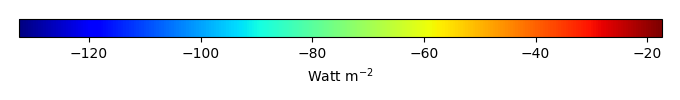MODEL MEANBIAS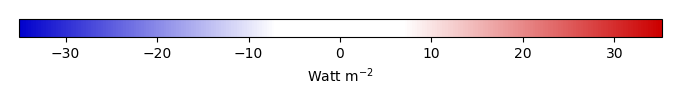BIAS SCORERMSE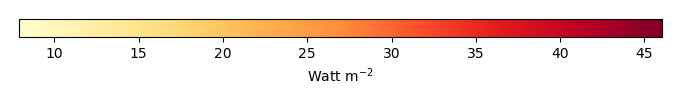RMSE SCOREBENCHMARK INTERANNUAL VARIABILITY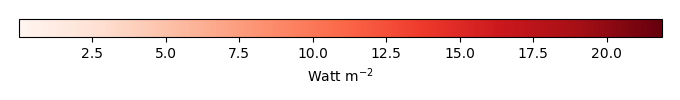MODEL INTERANNUAL VARIABILITYINTERANNUAL VARIABILITY SCOREBENCHMARK MAX MONTHMODEL MAX MONTHDIFFERENCE IN MAX MONTH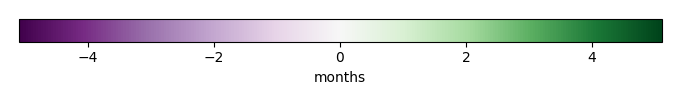SEASONAL CYCLE SCORESPATIAL TAYLOR DIAGRAMMODEL COLORS# Spatially integrated regional mean

MODEL COLORSREGIONAL MEANANNUAL CYCLEMONTHLY ANOMALYANNUAL CYCLE# All Models

BenchmarkCLM4CLM4.5CLM5# Data Information

creation_date: Tue Jul 1 08:25:00 PDT 2014

source_file: This product is generated from monthly 1 degree GEWEX SRB Radiation observations